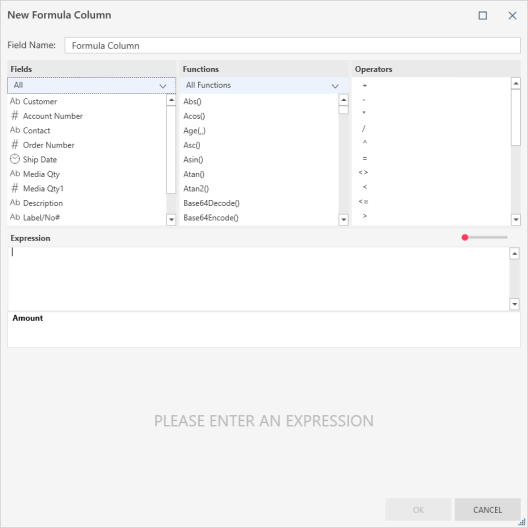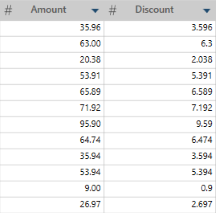Altair® Monarch®

# Creating New Formula Columns

### Description

Creates a new column based on a calculation.

### Steps

1. Right+Click on a column and select Column > New Formula Column from the options that display. The New Formula Column dialog displays:

2.3. Rename this column by replacing the name indicated in the Field Name field.

4. Define the expression for calculating the new field value:

• Select fields, operators, functions, and operators from the lists above the expression builder.

• Refer to functions reference for a list and description of all functions you can use within an expression.

•Note that:

• You can use the drop-down provided below the Functions column to specify a set of functions to display (e.g., Date, String, Numeric, Trigonometry, etc.).

• As you define the formula, a sample of the output is displayed in the preview area at the bottom of the dialog box.

5. Select OK to add the specified calculation as a new field to your table.

### Example

In this example, the first column (Amount) is the original column. Discount is a calculated field that shows the 10% discount value. It uses the following simple formula: Amount * .10:Other expressions can look like this:

• If(Customer="Ace Resellers",Unit_price*.55, Unit_price*.65)

• FiscalWeek([Ship Date])

• Replace(Contact,"Smith","Smith-Jones")